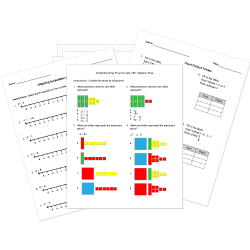Looking for Algebra worksheets?
Check out our pre-made Algebra worksheets!
 Tweet##### Browse Questions
• Arts (659)
• English Language Arts (3081)
• English as a Second Language ESL (1429)
• Health and Medicine (722)
• Life Skills (476)
• Math (2437)

• ### Systems of Equations

• #### Trigonometry

• Physical Education (420)
• Science (6734)
• Social Studies (2161)
• Study Skills and Strategies (13)
• Technology (485)
• Vocational Education (1000)

You can create printable tests and worksheets from these Grade 10 Sequences and Series questions! Select one or more questions using the checkboxes above each question. Then click the add selected questions to a test button before moving to another page.

Previous Next
What is the explicit function of the geometric sequence 3, 4.5, 6.75, ...?
1. $f(n)=1.5f(n-1)$ where $f(1)=3$
2. $f(n)=3*1.5^(n-1)$
3. $f(n)=1.5*3^(n-1)$
4. $f(n)=3f(n-1)$ where $f(1)=1.5$
Given the sequence $1,4,16,64,256,...$, which of the following correctly defines this sequence in a recursive form? Assume that $n in NN$.
1. $t(1) = 1; \ \ t(n) = 4t(n-1), \ n>1$
2. $t(1) = 4; \ \ t(n) = 4t(n-1), \ n>1$
3. $t(1) = 1; \ \ t(n) = 1/4 t(n-1), \ n>1$
4. $t(1) = 1; \ \ t(n) = 2^(2(n-1)), n>1$
Grade 10 Sequences and Series CCSS: HSF-IF.A.3
What are the next 2 numbers in the sequence $1, 5, 9...$?
1. $45,225$
2. $14,18$
3. $13,17$
4. $32,128$
Grade 10 Sequences and Series CCSS: HSF-IF.A.3
Grade 10 Sequences and Series CCSS: HSF-IF.A.3
What is the $n^(th)$ term of the arithmetic progression, $-3, -8, -13, ...$?
1. $2+5n$
2. $8+5n$
3. $2-5n$
4. $8-5n$
Grade 10 Sequences and Series CCSS: HSF-IF.A.3
Given the recursive function $f(1) = 3; \ \ f(n) = 2 * f(n-1), n>=2$ for a geometric sequence, which of the following functions describes the same geometric sequence?
1. $f(n) = 3*2^(n-1), n>=2$
2. $f(n) = 3*2^(n-1), n>=1$
3. $f(n) = 3*2^n, n>=1$
4. $f(n) = 3*2^n, n>=2$
Grade 10 Sequences and Series CCSS: HSF-IF.A.3